## MSC (Biotechnology) Final Year Model Papers

#### Top Model Papers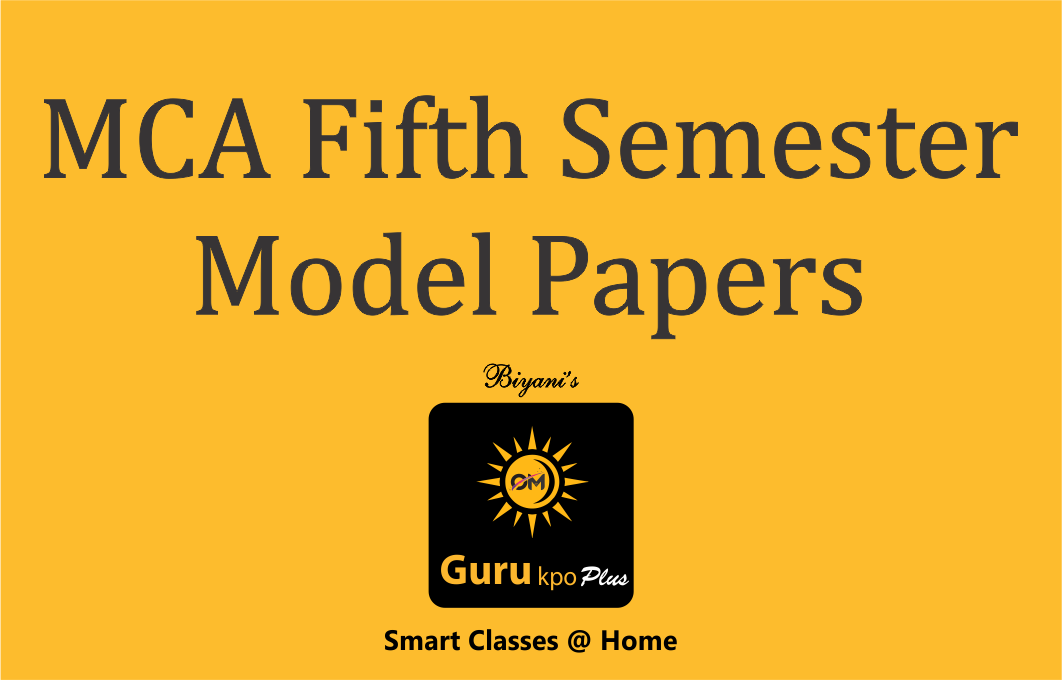#### MCA Fifth Semester Model Papers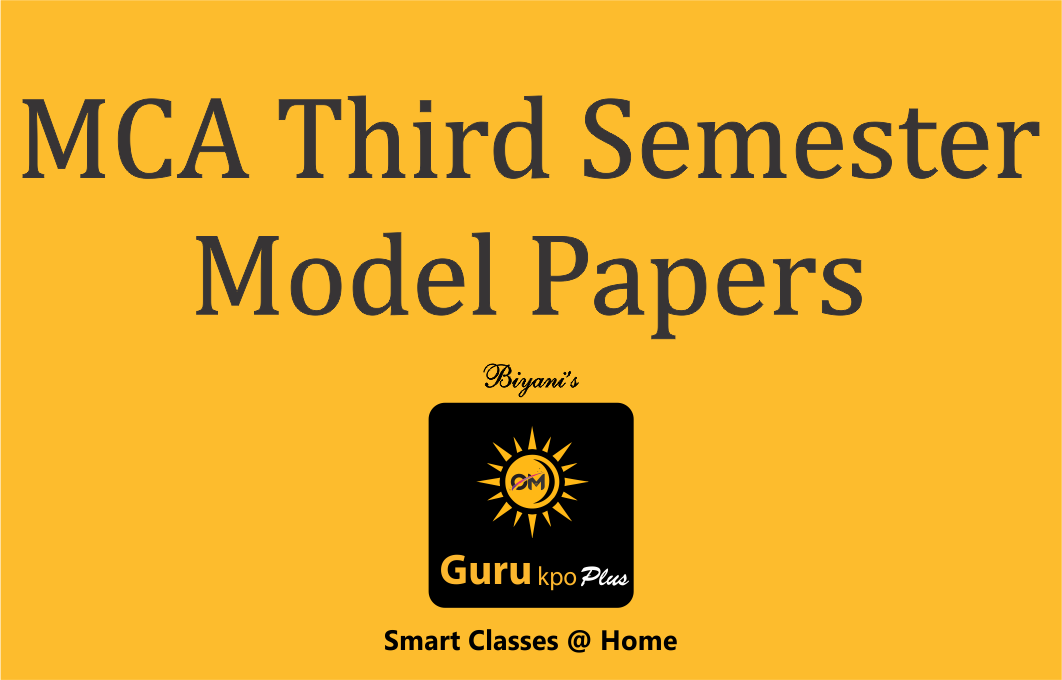#### MCA Third Semester Model Papers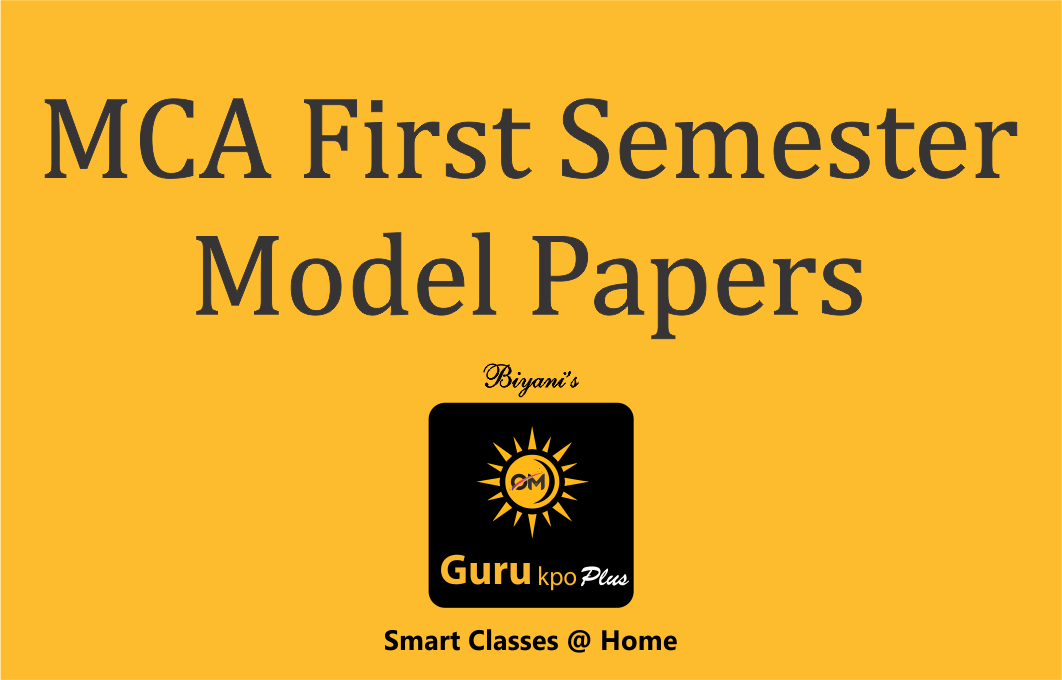#### MCA First Semester Model Papers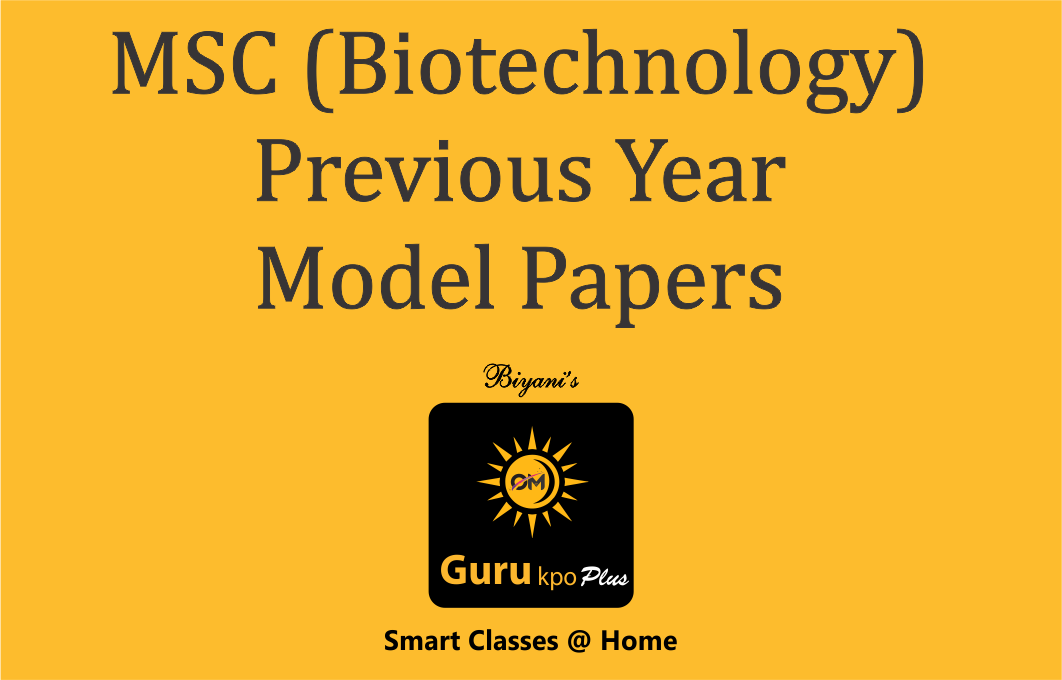#### MSC (Biotechnology) Previous Year Model Papers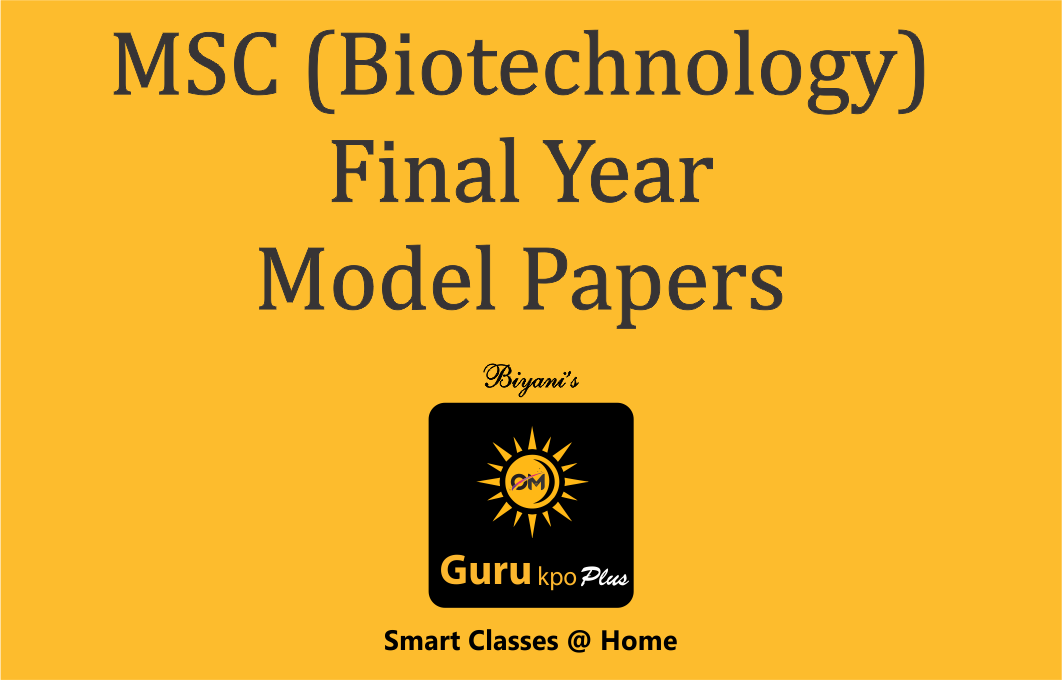#### MSC (Biotechnology) Final Year Model PapersSECTION-A
1. Introduction of Statistics : Growth of Statistics, Definition, Scope, Uses, Misuses and Limitation of Statistics, Collection of Primary & Secondary Data, Approximation and Accuracy, Statistical Errors.
2. Classification and Tabulation of Data : Meaning and Characteristics, Frequency Distribution, Simple and Manifold Tabulation.
3. Measuring of Central Tendency : Arithmetic Mean (Simple and Weighted), Median (including quartiles, decides and percentiles), Mode, Geometric and Harmonic Mean – Simple and Weighted, Uses and Limitations of Measures of Central Tendency.
SECTION-B
4. Measures of Dispersion : Absolute and Relative Measures of Dispersion; Quartile Deviation, Mean Deviation, Standard Deviation and their Coefficients; Uses and Interpretation of Measures of Dispersion.
5. Skewness : Measures of Skewness.
6. Index Numbers : Meaning and Uses of Index Numbers, Simple and Weighted Price Index Numbers, Methods of Construction of Index Numbers, Average of Relatives and Aggregative Methods, Problems in Construction of Index Numbers, Selection of Variables, Base, Weight, Average and Formula; Fishers Ideal Index Number, Base Shifting, Splicing and Deflating.
SECTION-C
7. Correlation : Meaning and Significance, Scatter Diagram, Correlation Graph, Karl Pearson‘s Coefficient of Linear Correlation between two Variables in Grouped and Ungrouped Data by Direct and Shortcut Methods, Coefficient of Correlation by Spearman‘s, Rank Differences Method and Concurrent Deviation Method.
8. Linear Regression.
9. Presentation of Data, Diagrammes/Graphs of Frequency Distribution and Histograms Ratio Scale Graph.